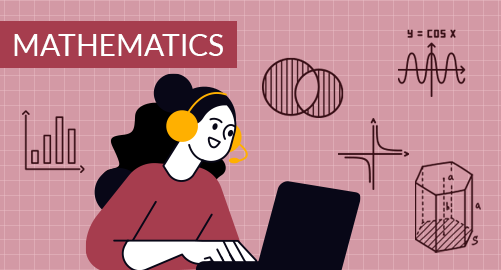#### through a demo today# Year 5 Mathematics

Mathematics
Year 5

The Year 5 Mathematics curriculum provides the students with foundational mathematical skills and concepts to prepare students for more advanced mathematical challenges. It covers various key areas, including numbers, factors and multiples, fractions, decimals, financial mathematics, introduction to algebra, geometry, measurements, data handling and probability. Students develop a deeper understanding of mathematical principles and problem-solving techniques, enhancing their ability to apply mathematics in real-life scenarios.

## What you'll learn

• 1

Number System

• 2

Factors and Multiples

• 3

Fractions and Decimals

• 4

Financial Mathematics

• 5

Introduction to Algebra

• 6

Geometry

• 7

Measurements

• 8

Data Handling and Probability

• 9

Time

## Skills you'll learnNumeracy Skills: Understanding numbers and their place values, mastering essential arithmetic operations, estimating resultsProblem-Solving Skills: Simplifying complex problems, recognising number patterns and relationships, applying divisibility testsArithmetic Operations: Basic arithmetic operations on fractions and decimals, real-world problem solving, rounding off and computational fluencyFinancial Literacy: Handling currency and exchanging the currency, understanding denominations, maintaining financial records, calculating discounts and percentages in real-world problemsAlgebraic Thinking: Understanding algebraic expressions and equations, recognising and applying the sequencesGeometrical Skills and Spatial Relationships: Proficiency in measuring angles, properties of 2D and 3D shapes, identifying 2D and 3D objects, recognising symmetrical figures in real lifeMeasurement Skills: Calculating the distance, finding the space occupied, understanding cardinal directions, conversions of unitsData Analysis and Predictions: Data organisation, create graphs, interpret data, calculate predictionsReasoning: Plan and schedule activities, estimate the time, understanding the passage of time